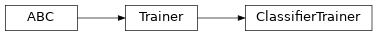ClassifierTrainer¶

Inheritance Diagramclass ashpy.trainers.classifier.ClassifierTrainer(model, optimizer, loss, epochs, metrics=None, callbacks=None, logdir=PosixPath('/home/docs/checkouts/readthedocs.org/user_builds/ashpy/checkouts/latest/docs/source/log'), global_step=None)[source]

ClassifierTrainer provide the standard training loop for a classifier.

Methods

 __init__(model, optimizer, loss, epochs[, …]) Instantiate the ClassifierTrainer trainer. call(training_set, validation_set[, …]) Start the training. train_step(features, labels) Train step.

Attributes

 ckpt_id_callbacks ckpt_id_global_step ckpt_id_model ckpt_id_optimizer ckpt_id_steps_per_epoch context Return the training context.
__init__(model, optimizer, loss, epochs, metrics=None, callbacks=None, logdir=PosixPath('/home/docs/checkouts/readthedocs.org/user_builds/ashpy/checkouts/latest/docs/source/log'), global_step=None)[source]

Instantiate the ClassifierTrainer trainer.

Parameters: model (tf.keras.Model) – A tf.keras.Model model. optimizer (tf.optimizers.Optimizer) – A tf.optimizers.Optimizer. loss (ashpy.losses.classifier.ClassifierLoss) – A loss function built following ashpy.executors`. epochs (int) – Number of training epochs. metrics (Union[Tuple[Metric], List[Metric], None]) – (Tuple/List): Tuple/List of ashpy.metrics.metric.Metric to measure on training and validation data. callbacks (List) – List of ashpy.callbacks.callback.Callback to to call on events logdir (str) – Checkpoint and log directory. global_step (Optional[py:class:tf.Variable]) – tf.Variable that keeps track of the training steps.

Examples

def toy_dataset():
inputs = tf.expand_dims(tf.range(1, 1000.0), -1)
labels = tf.expand_dims(
[1 if tf.equal(tf.math.mod(tf.squeeze(i), 2), 0) else 0 for i in inputs], -1
)
return tf.data.Dataset.from_tensor_slices((inputs, labels)).shuffle(10).batch(2)

model = tf.keras.Sequential(
[tf.keras.layers.Dense(10, activation=tf.nn.sigmoid), tf.keras.layers.Dense(2)]
)

loss = ClassifierLoss(tf.losses.SparseCategoricalCrossentropy(from_logits=True))
logdir = "testlog"
epochs = 2

if pathlib.Path(logdir).exists():
shutil.rmtree(logdir)

metrics = [
ClassifierMetric(tf.metrics.Accuracy()),
ClassifierMetric(tf.metrics.BinaryAccuracy()),
]

trainer = ClassifierTrainer(model=model,
optimizer=optimizer,
loss=loss,
epochs=epochs,
metrics=metrics,
logdir=logdir)
train, validation = toy_dataset(), toy_dataset()
trainer(train, validation)

shutil.rmtree(logdir)
Initializing checkpoint.
Starting epoch 1.
 Saved checkpoint: testlog/ckpts/ckpt-1
Epoch 1 completed.
Starting epoch 2.
 Saved checkpoint: testlog/ckpts/ckpt-2
Epoch 2 completed.
Training finished after 2 epochs.
_build_and_restore_models(dataset)[source]

Build and restore a Subclassed model by firstly calling it on some data.

_train_step[source]

Perform the training step using the distribution strategy.

call(training_set, validation_set, log_freq=10, measure_performance_freq=10)[source]

Start the training.

Parameters: training_set (tf.data.Dataset) – Training dataset. validation_set (tf.data.Dataset) – Validation dataset. log_freq (int) – Specifies how many steps to run before logging the losses, e.g. log_frequency=10 logs every 10 steps of training. Pass log_frequency<=0 in case you don’t want to log. measure_performance_freq (int) – Specifies how many steps to run before measuring the performance, e.g. measure_performance_freq=10 measures performance every 10 steps of training. Pass measure_performance_freq<=0 in case you don’t want to measure performance.
train_step(features, labels)[source]

Train step.

Parameters: features – Input features. labels – The labels. Loss value.